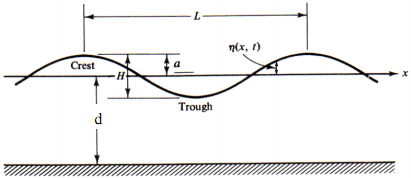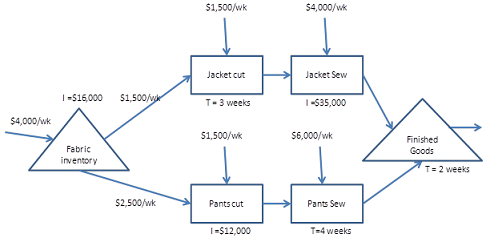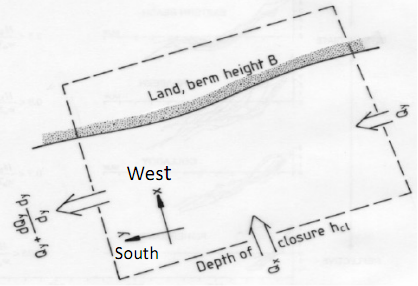### Write matlab codes to determine the wave characteristics

Assignment Help MATLAB Programming
##### Reference no: EM131057630

Question 1:

A wave with the deep-water characteristics of (T = 6.8 s and H = 5.0 m) is propagating toward a coast, where the water depth is 6.2 meters. At the coast, the surface elevation can be described as

η(t) = (H/2) cos(ω/t), and

the horizontal and vertical water particle velocities of the wave can be calculated with

u(t) = (ωH/2)(cosh(k(z+d))/sinh(kd))cos(ωt)

v(t) = -(ωH/2)(sinh(k+(z+d))/sinh(kd))sin(ωt)

where g is the gravity, H is the wave amplitude, k is the wave number, d is the mean water depth, ω is the angular frequency, z is the location below the mean water level (MWL).Write MATLAB codes to-

(i) Determine the wave characteristics (i.e. wave height, wave length, wave number, wave celerity) in deep water and at the coast (use the Dispersion relationship and fzero function in MATLAB);

(ii) Calculate the horizontal and vertical water particle velocities for t varies for 2 wave periods and z varies from 0 to -d (with an interval of 1 m).

(iii) Generate figures to present the time series (t varies from 0 to 2T) of the water particle velocities at the locations of the trough, z = -H/2 m and at the bottom. (iii) present the vertical profile of the magnitude of horizontal water particle velocity from the MWL to the sea bottom.

(iv) generate a similar graph as below for one wave period and at the mean  water level, to show the relationship between the surface elevation, the horizontal and vertical  water particle velocities.Question 2-

Consider a 5km stretch of coast oriented in the north-south direction with the ocean to the east. The predominant wave direction is from the east-south-east. At the southern end the typical breaker height is 1.4 m and the breaker angle is 10o. At the northern end, the breaker height is 1.45m and the breaker angle is 12o. The breaker parameter γb = 0.8.

The beach profiles along the section are similar with slopes near the break point of 1/40. The sand is made of quartz (s = 2.63, p = 0.28) with a median grain size of 0.22mm and measureable seasonal bed level changes are restricted to depths less than 6 metres. The berm height is 3 m AHD.The average shorenormal sediment transport rates (Qx) for 1993-2015 were saved in the data file "sediment.xlsm". There are no sinks and sources for sediment transport in the control domain. The erosion rate (metres of shoreline retreat rate) can be calculated using (see details in Coastal Process module lecture notes)

(1-p)(hc + B) ∂xs/∂t = -Qx + ∂Qy/∂y + Qsink - Qsource

Qy = (K/16(s-1)√γ) √gHb5/2 sin2θb

where

p is the sediment porosity,

xs is the shoreline coordinate

Qsource is a sediment input (e.g. river discharge, beach nourishment)

Qsink is a sediment loss (e.g. dredging)

K ≈ 0.77 is an empirical coefficient which has a weak dependence on grain size.

s is the specific weight

Hb is the breaker height

γ is the breaker index

θb is the wave crest angle at the break point

all other variables are as defined in the figure.

The aerial photographs of the stretch of the coast from 1993 to 2015 indicate the shoreline location, xs which were saved in the file "shoreline.xlsm".

Use Matlab to develop a numerical model to calculate the location of the shoreline from 1993 to 2015, i.e. xs. (assume xs = 0 in 1993)  Evaluate the accuracy of the model.

Attachment:- Assignment.rar

#### Plot the pole-zero pattern, draw curves of m(w) versus

Use Matlab to check your work (pzmap). Also, use Matlab to create the Bode plot (use the “bode” command.) If this circuit were used as a filter, what type of filter would it b

#### Use matlab to verify the central limit theorem

Use Matlab to verify the central limit theorem for the sum of N independent exponential RVs (with a=0 and b=2) for N= 10 and 20. Repeat for the sum of N independent Weibull

#### Write a user defined function

Write a user defined function FindFrequency which inputs are a time and a wave elevation vector and output the approximated wave frequency (fappr=FindFrequency(t,eta)).

#### Evaluate the function at a range of x values

Write a function file, that accepts values of x as inputs and outputs the value of y when the input is passed through the following function. Evaluate the function at a range

#### Simulate using matlab a casino style game

Your assignment requires you to simulate, using Matlab, a casino style game of chance. In theory these games are completely governed by the rules of probability and should b

#### Design the circuit and select the appropriate components

ENG318 Project - Design the circuit and select the appropriate components for that circuit to fulfill the requirements of the device and Verify your design by MATLAB simulatio

#### Write a function that takes a list of integers

Using ML write a function that takes a list of integers as argument and returns a pair consisting of the sum of the even position and the sum of the odd positions of the list.

#### How much did he spend each day

A hungry college student goes to the cafeteria and buys lunch. The next day he spends twice as much. The third day he spends \$1 less than he did the second day. At the end o

### Write a Review